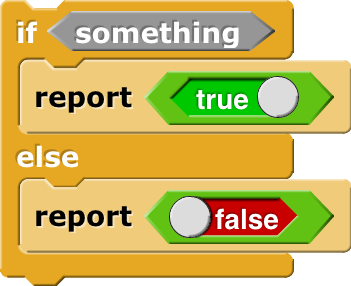# Math Versus Computers -- Year-Long Project

Hello everyone,

For my senior year in high school, I've been creating computer (using Snap!) and mathematical solutions to better understand the differences between the two approaches and to see what we can gain from using either medium. I'm posting a sample of what I've done and would appreciate any feedback on it.

Thank you,
Misha

Where's the project?

also, it's written Snap!
(except when it's written Snap!)

I. The Lehmer Sieve is an impressive device that implemented pulleys and ropes of varying lengths to factorize numbers as large as:

293 +1=335259109397158278832903110321

...in as quickly as 3 seconds!

The algorithm searches for an(x,y)pair of integers that satisfiesn=x2+y2 ②, where n is the target number. Hence: n=(x-y)(x+y) ① where each bracketed term (x-y)and(x+y) is a factor ofn. (Difference of Squares); (Given)

II. Solving for y in equation ① yields:

1.n=x2+y2 ①

1. y2=x2-n

2. y=x2-n ③

III. We obtain a lower bound of: xn>(closest perfect square ton)

Because y=x2-n ③ and y and x must be integers (Given)x2-n must equal an integer, or a perfect square, because ymust be an integer. Thus, the smallest value x can be is the closest perfect square of the target number (1), followed by the actual square root of the target number (2):

(1)

y=x2-n ③

y=(n)2-n y=n-n y=0=0

(2)

1. y=x2-n ③
2. Let n=4181 y=x2-4181
3. The closest perfect square of4181is 64; therefore, we have:x4181>64
4. Rightly so, the lower bound indicates that x must be an integer greater than 64 (i.e. 65, 66, 67...) as x=64 evaluate to an imaginary number (i):

(y=642-4181=4096-4181=-85=9.2195444... i)

IV. x=75 is the first integer that satisfies the parameters

For x={64, 65, 66, 67...}the first instance of x that satisfies the lower bound and evaluates to an integer in y=x2-n ③if n=4181 is x=75

y=x2-n ③ y=752-4181 y=38

V. Factors are: 37 and 113

n=(x-y)(x+y) ①

Factor 1:(x-y)(75-38)=37

Factor 2:(x+y)(75+38)=113

Therefore, the factors of 4181 are:{37,113}

Verifying the solution:n=(x-y)(x+y) ① 4181=(37)(113) True!

VI. Factors {37, 113} are prime, where 113 is the largest prime factor

Choice of prime number test Fermat’s Little Theorem:

If (ap-a) mod p=0 ④, for a{1, 2, ... , p-1} then number pprime. Repeat to increase confidence in the primality of p, the target number. So, some calculations that follow:

Let a=1

It follows: (1113-1)mod 113=0 0 mod 113=0 True!

Let a=2

It follows: (2113-2) mod 113=0 (1.038459371707E+34) mod 113=0 True!

Let a=3

It follows: (3113-3) mod 113=0 (1.038459371707E+34) mod 113=0 True!

Conclusion: Of course, this test could continue indefinitely, and I’m obtaining increasingly large numbers so I will stop here (before my calculator breaks) but at this point, I’m pretty confident that 113 is a prime number. 113 is also the largest prime factor of 4181 because of the lower bound obtained in III and abiding by that lower bound and approaching the minimum number (first instance) where its true, we should get the maximum and minimum factors where 113 is clearly the maximum.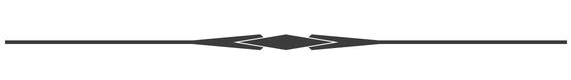Citations:

Kyle Miller (https://math.stackexchange.com/users/172988/kyle-miller), How to find prime factors of big numbers made up of big prime factors?, URL (version: 2017-09-26): https://math.stackexchange.com/q/2445525

Well... That sounds very powerful. Computers can't do that because the machine has so many interactions between ropes, that computers can't build (without slowing it down to 2^n)?
Is this what allows quantum computers escape?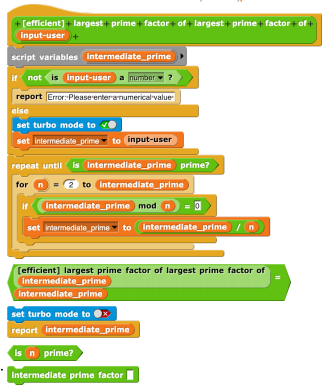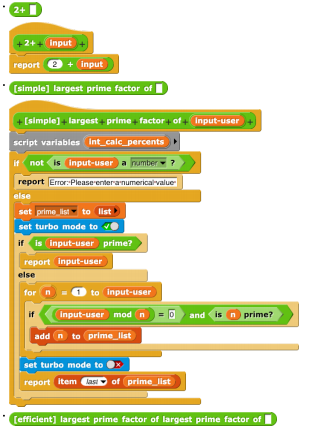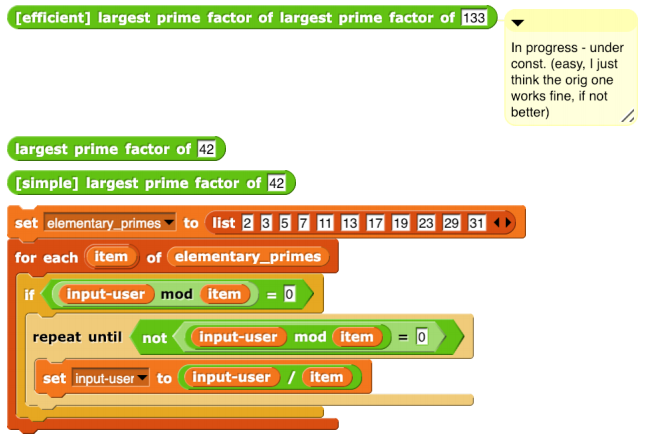Hi, Misha! Nice to have a mathematician around.

The project looks great. I'm imagining a visualization to go with it, with sprites for ropes and pulleys!

You may be interested to hear that we are seeking funding to add a computer algebra system to Snap!, so you could build this sort of project more straightforwardly.

I have a very small critique of your code. Wherever you say